# Y and Delta Resistor Network Calculations

Because of its shape, the network shown in Figure 47 is called a T (tee) or Y (wye) network.

These are different names for the same network.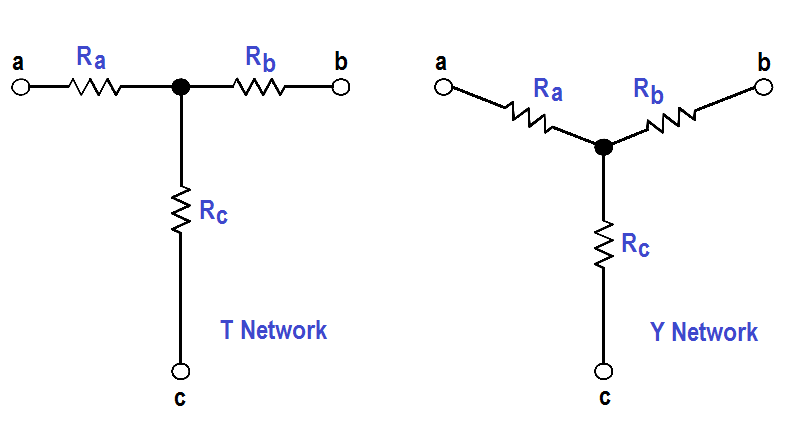Figure 47 : T or Y Network

The network shown in Figure 48 is called π (pi) or ∆ (delta) because the shapes resemble Greek letters π and Ω. These are different names for the same network.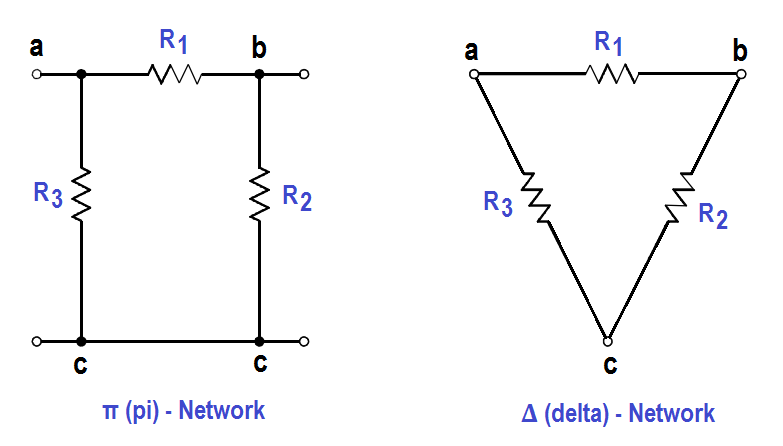Figure 48 : π (pi) or ∆ (delta) Network

In order to analyze the circuits, it may be helpful to convert Y to ∆,or ∆ to Y, to simplify the solution. The formulas that will be used for these conversions are derived from Kirchhoff’s laws.

The resistances in these networks are shown in a three-terminal network. After we use the conversion formulas, one network is equivalent to the other because they have equivalent resistances across any one pair of terminals (Figure 49).

∆ to Y conversion:Rule 1: The resistance of any branch of a Y network is equal to the product of the two adjacent sides of a ∆ network, divided by the sum of the three ∆ resistances.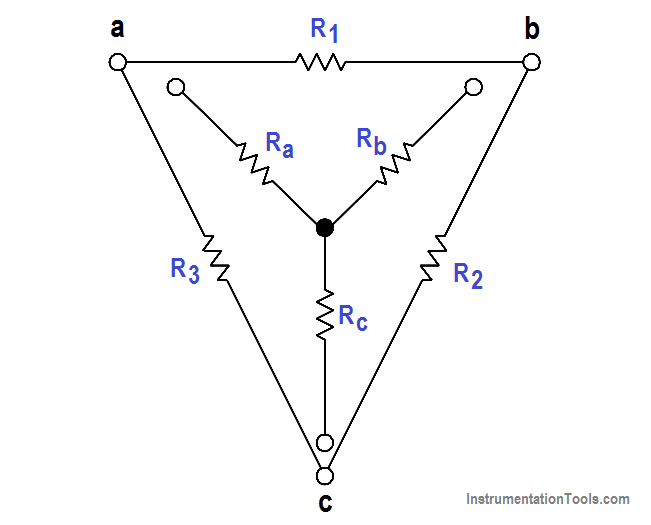Figure 49 : Y – ∆ Equivalent

Y to ∆ conversion: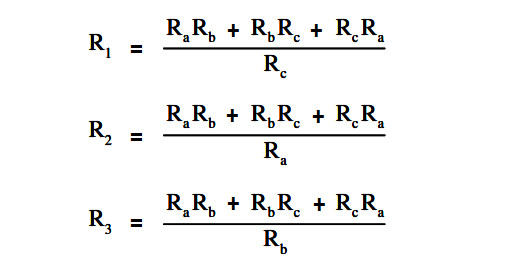Rule 2: The resistance of any side of a ∆ network is equal to the sum of the Y network resistance, multiplied in pairs, divided by the opposite branch of the Y network.

Let us consider a bridge circuit (Figure 50).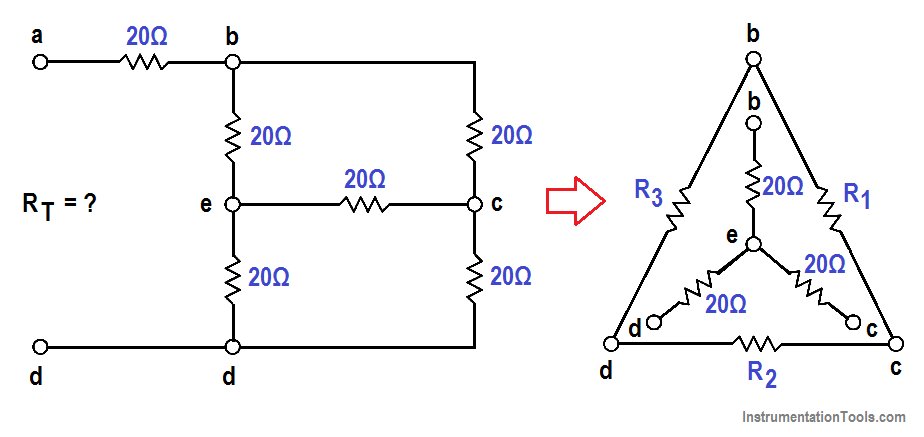Figure 50 : Bridge Circuit

Find Rt at terminals a and d.

Step 1: Convert the Y network (b-e, e-c, e-d) to the equivalent ∆ network.

Using Rule 2:

R1 = { (20×20) + (20×20) + (20×20) } / 20 = 1200/20 = 60Ω

R2 = 1200/20 = 60Ω

R3 = 1200/20 = 60Ω

Step 2: Now, we can redraw the Y circuit as a ∆ circuit and reconnect it to the original circuit (Figure 51):Figure 51 : Y – ∆ Redrawn Circuit

Step 3: Reduce and simplify the circuit. Note that the 20Ω and 60Ω branches are in parallel in Figure 51. Refer to Figures 51 and 52 for redrawing the circuit in each step below.

Rp = (R1R4) / (R1 + R4) = (20×60) / (20+60) = 1200/80 = 15Ω

Rq = (R1R5) / (R1 + R5) = (20×60) / (20+60) = 1200/80 = 15Ω

Rr = [ R3(Rp + Rq) ] / [R3 +Rp+Rq] = [60x(15+5)] / [60+30] = 1800/90 = 20Ω

RT = 20 + 20 = 40ΩFigure 52 : Steps to Simplify Redrawn Circuit